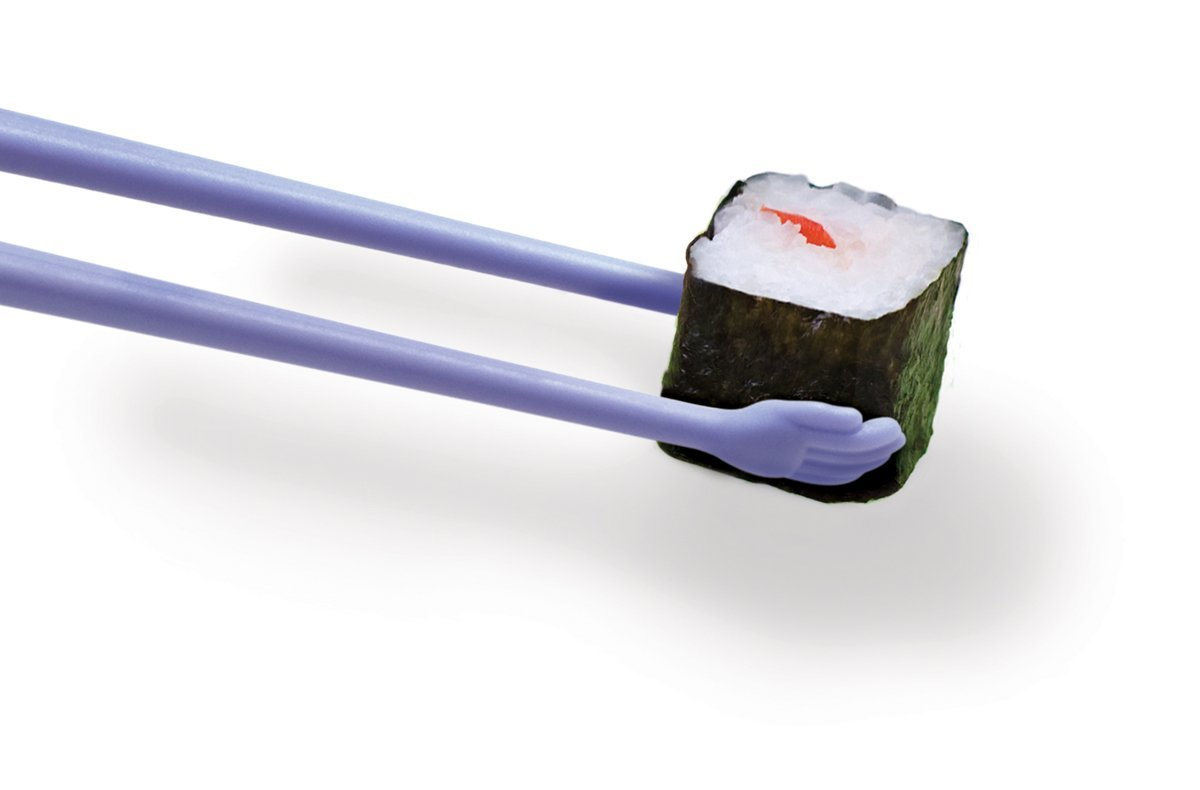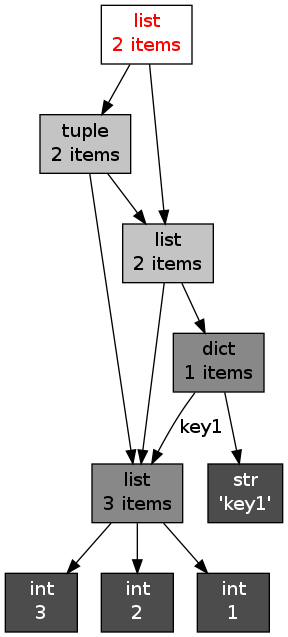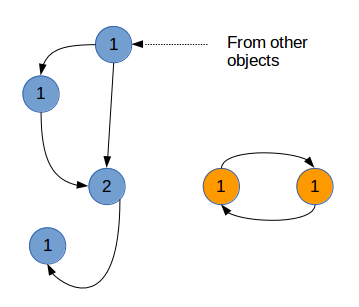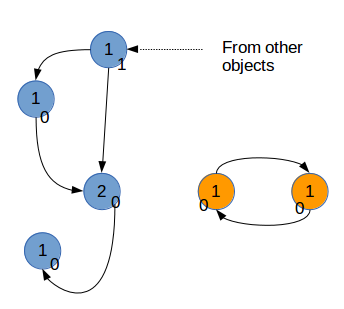### python gc_python,gc

a = 1a = 1

print(id(a))print(hex(id(a)))

11246696

‘0xab9c68’

a = 1b= 1

print(id(a))print(id(b))

11246696

11246696#True

a = 1b= 1

print(a isb)#True

a = “good”b= “good”

print(a isb)#False

a = “very good morning”b= “very good morning”

print(a isb)#False

a =[]

b=[]print(a is b)from sys importgetrefcount

a= [1, 2, 3]print(getrefcount(a))

b = a

print(getrefcount(b))Python的一个容器对象(container)，比如表、词典等，可以包含多个对象。实际上，容器对象中包含的并不是元素对象本身，是指向各个元素对象的引用。classfrom_obj(object):def __init__(self, to_obj):

self.to_obj=to_obj

b= [1,2,3]

a=from_obj(b)print(id(a.to_obj))print(id(b))from sys importgetrefcount()

a= [1, 2, 3]print(getrefcount(a))

b=[a, a]print(getrefcount(a))x = [1, 2, 3]

y= [x, dict(key1=x)]

z=[y, (x, y)]importobjgraph

objgraph.show_refs([z], filename=’ref_topo.png’)objgraph是Python的一个第三方包。安装之前需要安装xdot。

sudo apt-get install xdot

sudo pip install objgraph

a =[]

b=[a]

a.append(b)

a =[]

a.append(a)print(getrefcount(a))from sys importgetrefcount

a= [1, 2, 3]b=aprint(getrefcount(b))delaprint(getrefcount(b))del也可以用于删除容器元素中的元素，比如:

a = [1,2,3]

del a

print(a)from sys import getrefcount

a = [1, 2, 3]

b=aprint(getrefcount(b))

a= 1

print(getrefcount(b)))。a = [1, 2, 3]del a

del a后，已经没有任何引用指向之前建立的[1, 2, 3]这个表。用户不可能通过任何方式接触或者动用这个对象。这个对象如果继续待在内存里，就成了不健康的脂肪。当垃圾回收启动时，Python扫描到这个引用计数为0的对象，就将它所占据的内存清空。

importgcprint(gc.get_threshold())

Python同时采用了分代(generation)回收的策略。这一策略的基本假设是，存活时间越久的对象，越不可能在后面的程序中变成垃圾。我们的程序往往会产生大量的对象，许多对象很快产生和消失，但也有一些对象长期被使用。出于信任和效率，对于这样一些“长寿”对象，我们相信它们的用处，所以减少在垃圾回收中扫描它们的频率。Python将所有的对象分为0，1，2三代。所有的新建对象都是0代对象。当某一代对象经历过垃圾回收，依然存活，那么它就被归入下一代对象。垃圾回收启动时，一定会扫描所有的0代对象。如果0代经过一定次数垃圾回收，那么就启动对0代和1代的扫描清理。当1代也经历了一定次数的垃圾回收后，那么会启动对0，1，2，即对所有对象进行扫描。

importgc

gc.set_threshold(700, 10, 5)

a =[]

b=[a]Python作为一种动态类型的语言，其对象和引用分离。这与曾经的面向过程语言有很大的区别。为了有效的释放内存，Python内置了垃圾回收的支持。Python采取了一种相对简单的垃圾回收机制，即引用计数，并因此需要解决孤立引用环的问题。Python与其它语言既有共通性，又有特别的地方。对该内存管理机制的理解，是提高Python性能的重要一步。